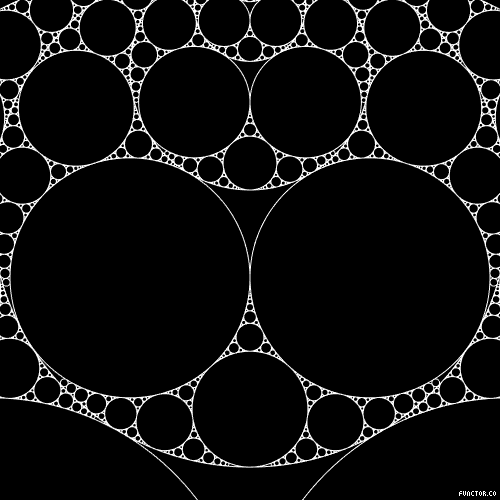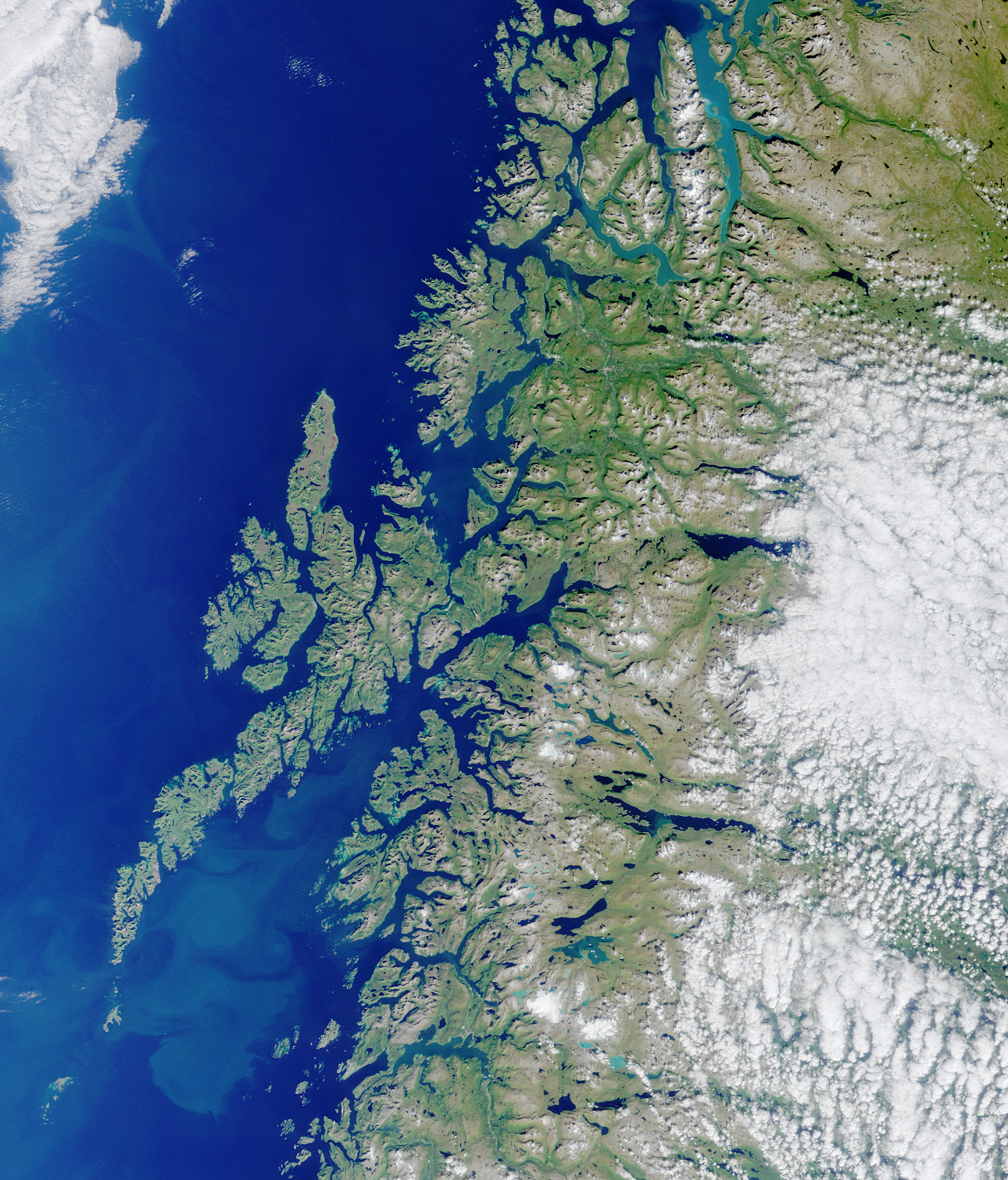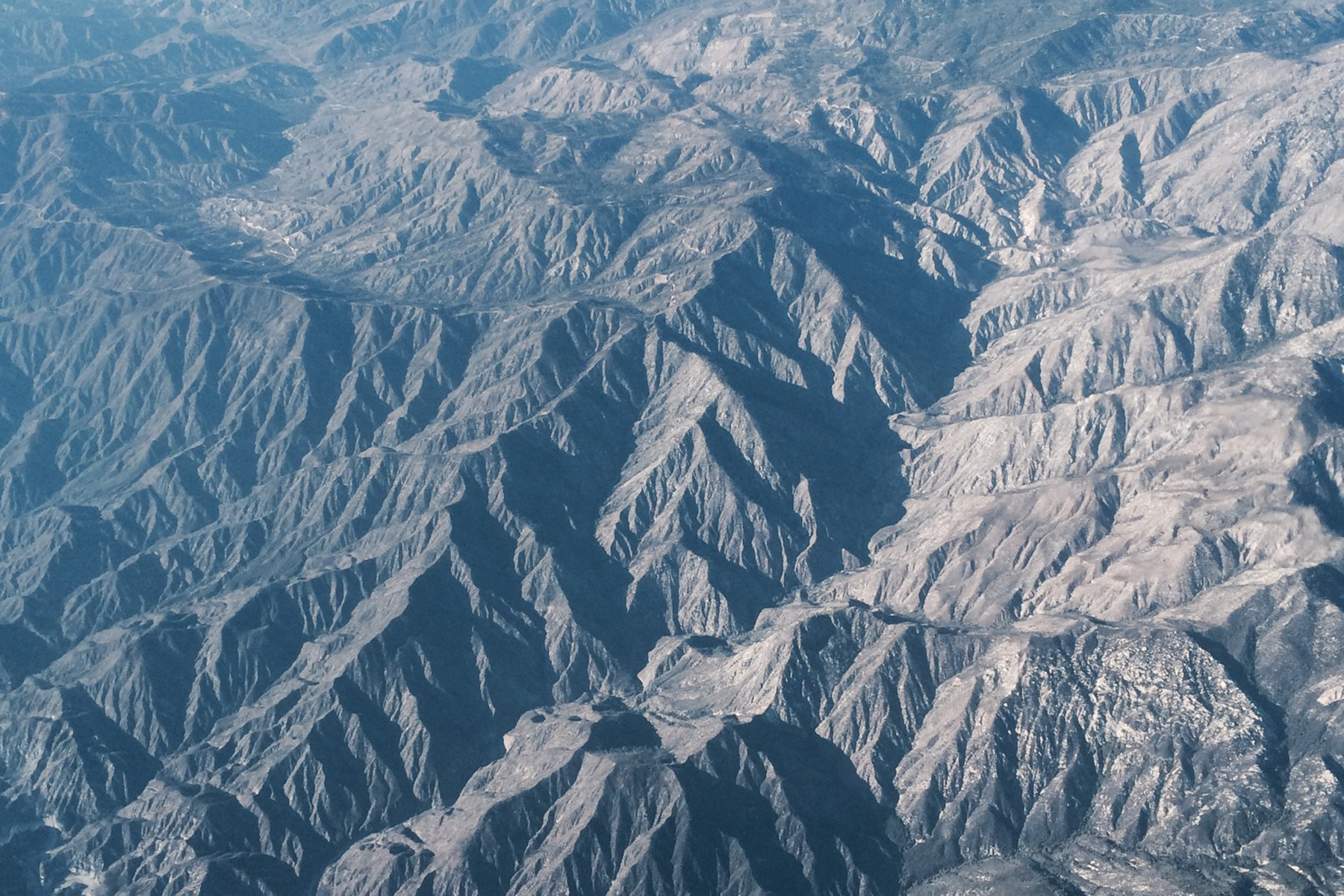## Fractal World

#### Alyssa Joines

##### What is a Fractal?

Fractals are multidisciplinary marvels. When most people hear the word "fractal" they might think of beautiful computer-generated images frequently used as desktop backgrounds, but they're found and used in a wide variety of subjects. Fractals are not only helpful in modeling chaos and in simulations of particle motion but also in technology, biology, and art. Approximate fractals are also found in many natural phenomena. To name just a few examples, fractal branching has been observed in lightning, leaves, trees and river networks. What defines a fractal can sometimes be unclear but they can be identified through properties such as Self-Similarity and Fractional Dimensions.

##### Self-Similarity

A fractal is typically a geometric pattern that repeats at infinite scales. This property is referred to as self-similarity. The idea of self-similarity is that if you were to take the fractal as a whole and then zoom in on one smaller part, you would see something very similar or identical to the whole. This means that fractals are composed of smaller versions of themselves. In this way, fractals are surprisingly very simple to construct despite their complex appearance. It’s also possible for a fractal to be self-affine, which is essentially a distorted self-similarity where smaller copies of the whole are each transformed differently instead of following a uniform set of rules.1

##### Fractal Dimension

Fractals are non-Euclidian geometries meaning they cannot be defined the same way that the geometries most people are familliar with are. While the topological dimensions with which we normally classify the line, square, and cube (1, 2, and 3, respectively) are the most familiar, there are also non-integer dimensions in which most fractals exist. For example the fractal dimension of the Koch snowflake is 1.26. This number can be used as a measure of the roughness of the geometry. While most fractals have non-integer dimensionality it's also possible for them to exist in an integer dimension if enough empty space exists within. For example, if there is an object that typically exists in 3 dimensions but just enough of its volume has been removed it will then measure as a 2 dimensional geometry. To find a fractal dimension there are multiple methods: one for fractals with defined self-similarity, and one more typically used on natural fractals called the box counting method.2##### Iterated Function Systems (IFS)

Iterated function systems are one method of constructing a self-similar fractal. These fractals are created using many versions of itself that have been transformed according to the function of that system. Each of those transformed copies are made up of more copies following the same transformation rules and so on and so on, making these fractals infinitely detailed. The different ways that the copies are transformed are through scaling, reflection, rotation, and translation (vertical or horizontal motion). Each IFS uses a different set of rules.3

##### Complex Numbers

Using computers, fractals can be created using complex numbers. The complex numbers in these fractals are made up of an x value which is the real component and a y value which is the imaginary component (both c and z are complex numbers). In Julia sets, the equation z = z² + c is used, where c is a constant complex number and z is found and then fed back into the formula after each iteration creating a set of points when |z| <2 that lie in the Julia set. By changing the constant c you can generate different Julia sets. The Mandelbrot set is similar and contains different Julia sets within it, acting essentially as a map of all them. It uses the same equation as the Julia set, except that c is another variable and not a constant. Values that diverge are not in the set. For example, if c = -1 it will be bounded and in the set, but if c = 1 it will be infinite/unbounded and not in the set.4

When creating these images the color is typically determined by how many iterations it takes z to “escape”, which is this case would mean to have a value greater than 2. The magnitude of iterations required to get the infinite detail in these sets is immense, which is why the Mandelbrot set was not discovered until there was access to computers.

Examples: Julia Set, Mandelbrot Set

•##### Koch Curve
••##### Levy C Curve
•##### Hilbert Curve
••##### Mandelbrot Set##### Where?

Fractals patterns and properties can be found in all parts of nature such as large scale terrain, plants, and water. Weather patterns have a fractal nature, as do lightning and drops of water. These drops of water fall from clouds as rain that may enter a stream which may carve out a mountain and then reach a cliff and break into a waterfall. All of these things occur in a fractal pattern, as is seen from many aerial views of mountains and coastal inlets and rivers. We see fractal properties across all scales, from bacteria growth and the organization of the chromatin in our cells, to our circulatory and pulmonary systems. Similarly, fractal branching is observed in trees and leaves and a large variety of other plants.

##### Why?

Why has everything in the last paragraph come to exist with fractal properties? There are many reasons why fractal organization is advantageous. The big one is efficiency (both energy efficent and spatially efficient). Fractal organization can allow a lot to be compacted into a very small space. For example, our cells must be organized to be as energy efficient and spacially efficient as possible so it makes sense that the chromatin in our cells would be organized using a fractal pattern. Seeing as our cells are microscopically small yet have to contain all of our genetic information along with organelles etc. theres definitely the need for this kind of organization. The branching seen in trees and our circulatory system are also built for efficiency. These fractal branching patterns allow easy, fast, and even distribution of blood or nutrients to all parts of the body or plant covering a large area without taking up valuable space.

••••••##### Do self-similar plants also have fractal dimensions?

The plant that I focused on is Brassica Oleracea which is more commonly known as wild cabbage. This plant is cultivated into a variety of vegetables including broccoli and cauliflower. Both broccoli and cauliflower are fractal in design, beautifully exhibiting the property of self-similarity. One head of broccoli or cauliflower can be divided into many smaller heads. To learn more about this interesting phenomena I decided to do a short lab. The purpose of this lab is to define the fractal dimensions of broccoli and cauliflower. The concept was inspired by a quote from Benoit Mandelbrot’s book The Fractal Geometry of Nature. He posed the question, "Could it be significant that part of the geometric difference between cauliflower and broccoli is quantified by a fractal dimension?"5 This led me to wonder what the fractal dimensions of the two vegetables are, and if this holds some kind of significance, especially considering the fact that both cauliflower and broccoli are derived from the same plant.

##### Procedure:
2. Measure length of the whole head
3. Break into the next decomposition (first level of smaller pieces that branch immediately from the whole) and measure length.
4. Record the measurements for each length and then take the mean length of all of the pieces from that decomposition.
5. Repeat for each level of branching
6. Calculate dimension for each level of branching using the equation: N = 1/(sd), where N is the number of pieces that branched off in each level and s is the scaling factor for those pieces. S can be found by dividing the mean length of the previous level by the mean length of the smaller level.6 (i.e. if the whole broccoli length measurement was 16 cm and the first level of branching mean length was 7.5 cm 16/7.5=2.13 so s = 1/2.13)
7. Solve for d for each decomposition
8. Take the mean of all of the values of d that you get for each level and that is the D value for that whole piece of broccoli or cauliflower
##### My Measurements
• ###### Decomposition 1
• N = 17
• s = 1/2.654
• 17 = 1/(1/2.654d)
• d1 = 2.90268
• ###### Decomposition 2
• N = 8
• s = 1/2.431
• 8 = 1/(1/2.431d
• d2 = 2.34092
• ###### Decomposition 3
• N = 6
• Too difficult to accurately measure length.
• ###### Decomposition 1
• N = 13
• s = 1/2.193
• 13 = 1/(1/2.193d)
• d1 = 3.26633
• ###### Decomposition 2
• N = 7
• s = 1/2.254
• 7 = 1/(1/2.254d
• d2 = 2.39436
• ###### Decomposition 3
• N = 11
• Too difficult to accurately measure length. Maintaining consistency with broccoli procedure.
###### Dfinal = 2.83

In this experiment I chose to take the mean of the lengths of each decomposition in order to find the "normal" length of each level of the broccoli and cauliflower. This is necessary because of the many irregularities that can result from the factors which influence plant growth. The is effective because of the Central Limit Theorem which tells us that given we have a large enough number of observations from a sample, the values will be distributed according to a normal distribution (a bell curve). Therefore taking the average of said values will result in a value which is representative of the whole.

##### What does the fractal dimension of an object tell us?

The main use of a fractal dimension is to quantify roughness of an object.7 Roughness can be defined as surface irregularity. When we’ve learned about dimension before, it has been in the sense of the number of possible ways to move on a plane or in space. For example, a 2-dimensional square lies on an (x,y) plane while a 3-dimensional cube lies on an (x,y,z) plane. Fractal dimensions, describe the relative amount of space that an object occupies. To reiterate, this means that a 3-dimensional object that has had a lot of its volume removed, or in other words lacks density, could have a fractal dimension of 2 because of the relative amount of space that object is actually occupying. This also means that there is a correlation between density and fractal dimension. An object that is very dense will have a higher fractal dimension than an object that is less dense. This is seen in the lab where we got Dbroccoli = 2.62 and Dcauliflower = 2.88. The larger value of D for the cauliflower shows that it is denser than the broccoli, which is confirmed by their measured dimensions.##### Applications

Hopefully by this point you are starting to realize that fractals are everywhere. While I chose to focus on broccoli and cauliflower, there are several fields of study and a variety of phenomena in which fractals are useful. They have an incredible ability to describe objects, events, and movements that may have previously been considered “random” or too complicated to define. Since fractals don’t follow the rules of Euclidian geometry, they’re effective in describing things in our physical world that couldn't previously be described.

That said, nature isn’t perfect and it has physical restrictions. For example, when you continue zooming in all the way to the cells that make up a tree’s branches or the molecules that make up a cloud, they obviously are not branch-shaped or cloud-shaped. These physical limits mean real fractal objects do not have the same infinite detail that computer-generated fractals do. Computer generated fractals and fractal organization are highly useful because of their infinite capabilities. Below are a handful of areas in which fractals and their amazing properties have been utilized.

##### More##### Brownian Motion
• Particle Motion
• Brownian Motion is essentially the way that particles move through a medium due to collisions. Previously believed to be random and directionless, it's been discovered that this motion is fractal over enough scales to be substantial. This can be useful in describing or predicting trajectories of particles.##### CGI Fractal Landscapes
• Computer-Generated Terrain
• As we now know, mountains are irregular fractal geometries. As a result of this knowledge, algorithms have been developed which generate fractal behavior to create realistic natural landscapes such as mountains. Computer-generated imagery (CGI) is used in movies, TV, and sometimes in printed media.##### Stock Market
• A Not-So-Random Walk Down Wall Street
• Many people might believe that the stock market is a world of randomness, but fractals beg to differ. The trends that exist in the stock market can be determined by fractals. By using fractals one can identify patterns within these trends allowing potential predictions.##### Art: Psychology and Forgeries
• Aesthetics & Fractals
• Fractals can be found in art and architecture throughout history, from Lonardo da Vinci to M.C. Escher. The presence of fractals in visual media has been shown to reduce stress levels and be generally appealing, a phenomenon referred to as fractal fluency. Not only are fractals soothing, but they have also shown to be helpful in identifying Jackson Pollock forgeries. Recent studies showed that fractal analysis helped identify forgeries with an impressive 93 percent success rate.##### The Internet
• Round-Trip Time and Connectivity Maps
• The above image is a cropped version of a spherical round-trip time (RTT) map created using the software called Walrus developed by Young Hong at the Center for Applied Internet Data Analysis. This software allows us to visualize connectivity and other internet-related data. RTT maps measure the time between when a query is sent and when a reply is received. Apart from the visual fractal qualities of these maps, the internet's structure itself is self-similar as it follows a power law distribution.##### The Universe?
• Theories of Fractal Cosmology
• The theories surrounding the formation and structure of our universe are varied. The universe does exhibit properties of self similarity and it varies in density. If one assumes that the universe is built upon the same chaotic dynamics that we observe in our physical world on earth, then a fractal universe would be the next logical assumption. However, there are a lot of critics of this theory, which is why I would suggest you follow the links and do some research to form your own opinion.